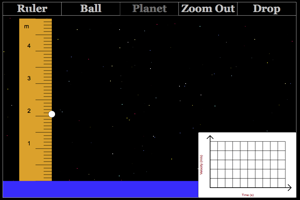Information About The Program
Acceleration Due To Gravity on a Planet (Lab)This lab was designed to have students test the factors that affect the acceleration due to gravity on a planet. Students will be able to modify the planet and then drop an object to determine the acceleration on the planet. As the object drops, it will display a velocity vs. time graph and students will find the slope of this graph to find the acceleration due to gravity on the planet.
Below are any Resources that go with this program